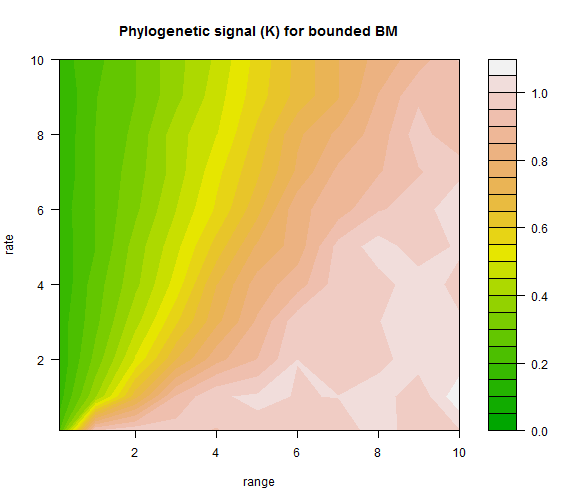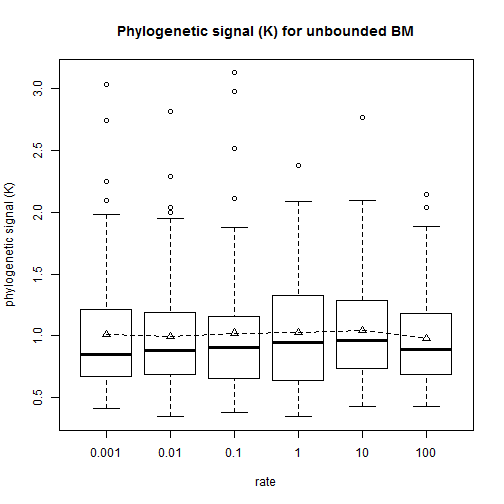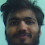## Tuesday, March 3, 2015

### Phylogenetic signal under Brownian evolution with bounds

A phytools user recently contacted me with some questions about her analyses and expressed some surprise at having discovered that bounds on the Brownian motion process tended to decrease phylogenetic signal measured using Blomberg et al.'s (2003) K statistic.

K is a measure of phylogenetic signal that is based on a standardized variance ratio - measuring the variance among vs. within clades compared to the ratio expected under Brownian motion. Consequently it has an expected value of 1.0, although the variance for a single simulation condition can be quite high.

Based simply on the logic of what K measures it shouldn't be too difficult to predict what happens to K when bounds are added to the Brownian process. That is, bounds will tend to cause the phenotypic values of species in unrelated clades to be more similar than expected under a pure Brownian process, making variability structured increasingly equally between & among clades. This effect should increase for decreasing bounds, as well as for increasing rate (σ2 of the Broanian process), because both will cause evolving lineages to reach the boundaries more often.

This is also pretty easy to demonstrate via simulation. Here I have just simulated under a range of different conditions for the rate & bounds given a single tree, but the results hold generally.

``````## load packages
library(phytools)
## function to conduct simulation
foo<-function(tree,sig2,range,nsim){
X<-fastBM(tree,a=range/2,sig2=sig2,bounds=c(0,range),nsim=nsim)
mean(apply(X,2,phylosig,tree=tree))
}
## simulate tree
tree<-pbtree(n=26,tip.label=LETTERS,scale=1)
## set simulation conditions
sig2<-c(0.1,1:10)
range<-c(0.1,1:10)
nsim<-200
## simulate
K<-sapply(sig2,function(s2,tr,r,n) sapply(r,foo,tree=tr,sig2=s2,
nsim=n),tr=tree,r=range,n=nsim)
colnames(K)<-sig2
rownames(K)<-range
## plot the result
filled.contour(x=range,y=sig2,K,xlab="range",ylab="rate",
zlim=c(0,max(K)),color.palette=terrain.colors,
main="Phylogenetic signal (K) for bounded BM")
``````Here we can see easily that for increasing rate (vertical axis) and decreasing bounds (horizontal axis), phylogenetic signal decreases - just as we predicted.

Note that, just as we showed in Revell et al. (2008; Syst. Biol.) there is no relationship between σ2 and phylogenetic signal, K for unbounded Brownian motion:

``````foo<-function(tree,sig2,nsim){
X<-fastBM(tree,sig2=sig2,nsim=nsim)
apply(X,2,phylosig,tree=tree)
}
sig2<-c(0.001,0.01,0.1,1.0,10,100)
K<-sapply(sig2,foo,tree=tree,nsim=100)
colnames(K)<-sig2
boxplot(K,xlab="rate",ylab="phylogenetic signal (K)",
main="Phylogenetic signal (K) for unbounded BM")
lines(colMeans(K),lty="dashed")
points(colMeans(K),pch=24)
``````That's it.

#### 1 comment:

1.Hi Liam,

Is there a way to fit Brownian motion with say reflecting or absorbing boundary conditions? I suspect one needs an expression for the vcv matrix, but I am limited by my math abilities to figure if its possible and how?

And thanks for this very useful resource!
Saurabh

Note: due to the very large amount of spam, all comments are now automatically submitted for moderation.